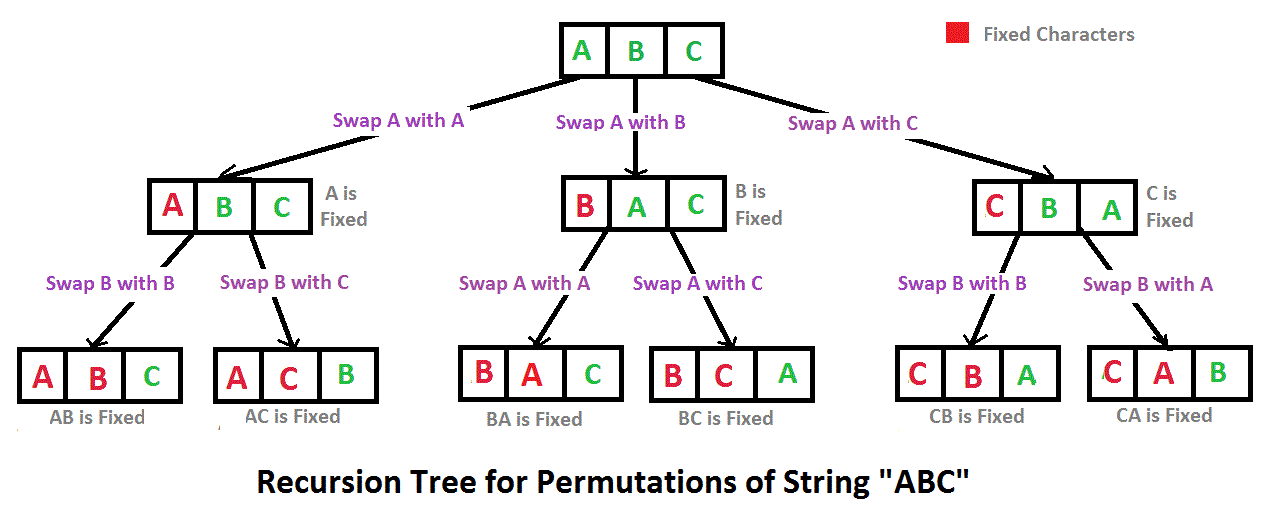Open in App
Not now

# C++ Program To Print All Permutations Of A Given String

• Last Updated : 11 Dec, 2021

A permutation also called an “arrangement number” or “order,” is a rearrangement of the elements of an ordered list S into a one-to-one correspondence with S itself. A string of length n has n! permutation.

Source: Mathword(http://mathworld.wolfram.com/Permutation.html)

Below are the permutations of string ABC.
ABC ACB BAC BCA CBA CAB

Here is a solution that is used as a basis in backtracking.## C++

 `// C++ program to print all permutations  ` `// with duplicates allowed  ` `#include   ` `using` `namespace` `std;  ` ` `  `// Function to print permutations  ` `// of string  ` `// This function takes three parameters:  ` `// 1. String  ` `// 2. Starting index of the string  ` `// 3. Ending index of the string.  ` `void` `permute(string a, ``int` `l, ``int` `r)  ` `{  ` `    ``// Base case  ` `    ``if` `(l == r)  ` `        ``cout<

Output:

```ABC
ACB
BAC
BCA
CBA
CAB```

Time Complexity: O(n*n!) Note that there are n! permutations and it requires O(n) time to print a permutation.

Auxiliary Space: O(r – l)

Note: The above solution prints duplicate permutations if there are repeating characters in the input string. Please see the below link for a solution that prints only distinct permutations even if there are duplicates in input.
Print all distinct permutations of a given string with duplicates.
Permutations of a given string using STL

Another approach:

## C++

 `// C++ program to implement ` `// the above approach ` `#include ` `#include ` `using` `namespace` `std; ` ` `  `void` `permute(string s,  ` `             ``string answer) ` `{ ` `    ``if``(s.length() == 0) ` `    ``{ ` `        ``cout << answer << ``"  "``; ` `        ``return``; ` `    ``} ` `    ``for``(``int` `i = 0;  ` `            ``i < s.length(); i++) ` `    ``{ ` `        ``char` `ch = s[i]; ` `        ``string left_substr = s.substr(0, i); ` `        ``string right_substr = s.substr(i + 1); ` `        ``string rest = left_substr + right_substr; ` `        ``permute(rest , answer+ch); ` `    ``} ` `} ` ` `  `// Driver code ` `int` `main() ` `{ ` `    ``string s; ` `    ``string answer = ``""``; ` ` `  `    ``cout << ``"Enter the string : "``; ` `    ``cin >> s; ` ` `  `    ``cout <<  ` `    ``"All possible strings are : "``; ` `    ``permute(s, answer); ` `    ``return` `0; ` `} `

Output:

```Enter the string : abc
All possible strings are : abc  acb  bac  bca  cab  cba```

Time Complexity: O(n*n!) The time complexity is the same as the above approach, i.e. there are n! permutations and it requires O(n) time to print a permutation.

Auxiliary Space: O(|s|)

Please refer complete article on Write a program to print all permutations of a given string for more details!

My Personal Notes arrow_drop_up
Related Articles